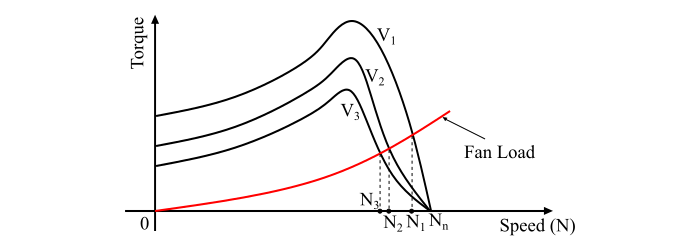# Speed Control of Induction Motor by Stator Voltage Control

The torque developed by the induction motor is given by,

$$\mathrm{\tau_𝑑 =\frac{𝑘 𝑠 𝐸_{20}^2 𝑅_2}{𝑅_2^2 + 𝑠^2𝑋_{20}^2} … (1)}$$

Where,

• k = constant of proportionality,
• s = fractional slip,
• E20 = per phase induced EMF in rotor at standstill,
• R2 = rotor circuit resistance, and
• X20 = reactance per phase of the rotor at standstill

And, the value of slip corresponding to maximum torque is given by,

$$\mathrm{𝑠_𝑚 =\frac{𝑅_2}{𝑋_{20}}… (2)}$$

The speed of a 3-phase induction motor can be varied by changing the supply voltage. Eqn. (1) shows that the torque developed in the motor is proportional to the square of the supply voltage and eqn. (2) shows that the slip corresponding to maximum torque is independent of the supply voltage. Also, the change in the supply voltage does not alter the synchronous speed of the motor. The figure shows the torque-speed characteristics of the 3-phase induction motor for varying supply voltage.The speed control of a three phase induction motor is obtained by changing the supply voltage until the torque required by the load is developed at the desired speed. The torque developed by the induction motor is directly proportional to the square of the supply voltage and the current is proportional to the voltage. Hence, when the supply voltage is decreased to reduce the speed for the same current, the torque developed by the motor is reduced. Therefore, the stator voltage control method is suitable for applications where the load torque decreases with the speed, as in the case of a fan load.

By the stator voltage control method, the speed of the motor can be varied within a small range. As the operation of the motor at voltages higher than the rated voltage is not permissible, thus this method allows the speed control only below the rated speed of the motor.

The speed control by the stator voltage control method is more suitable where intermittent operation of the motor is required. This method is also suitable for the speed control of the induction motors driving fans or pumps where the load torque varies as the square of the speed, since these motor drives require low torque at low speeds and this can be achieved with lower applied voltage without excessive motor current.The variable voltage for speed control of three phase induction can be obtained by using thyristor voltage controllers. Here, three pairs of back-to-back connected thyristors are required, one pair in each phase (see the circuit diagram). Each pair of the thyristors controls the voltage of the phase to which it is connected. The speed control of the motor is obtained by varying the conduction period of the thyristors.

Important – For low power rating operations, the back-to-back thyristor pair in each phase can be replaced by a triac.